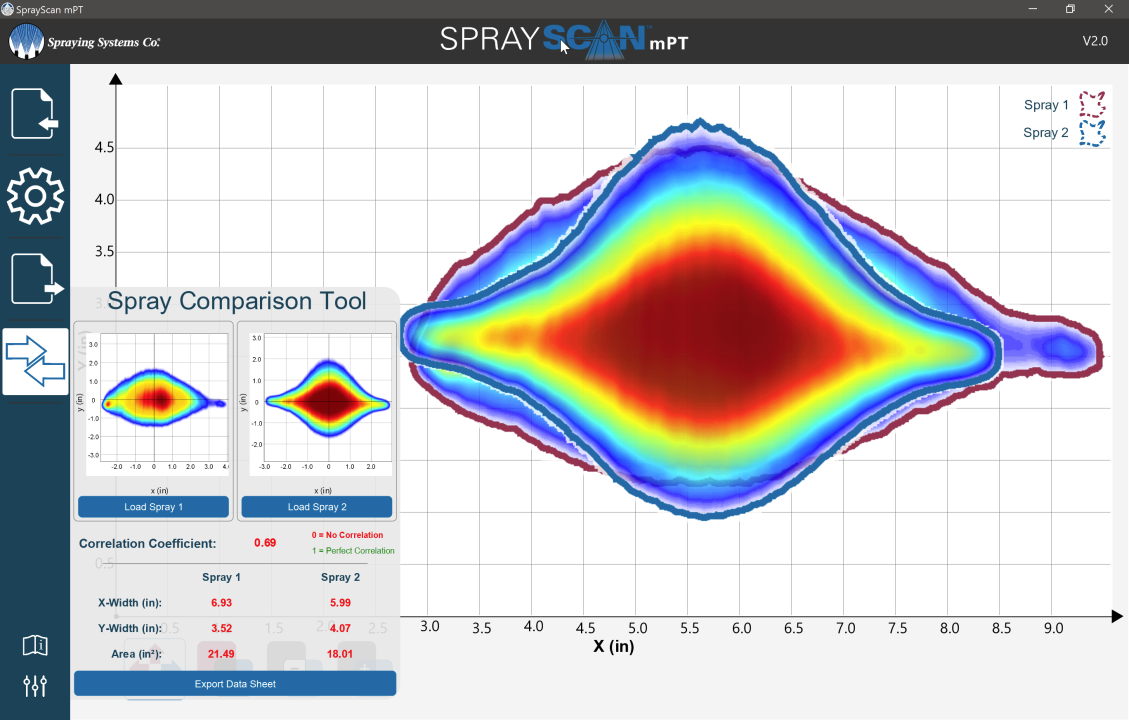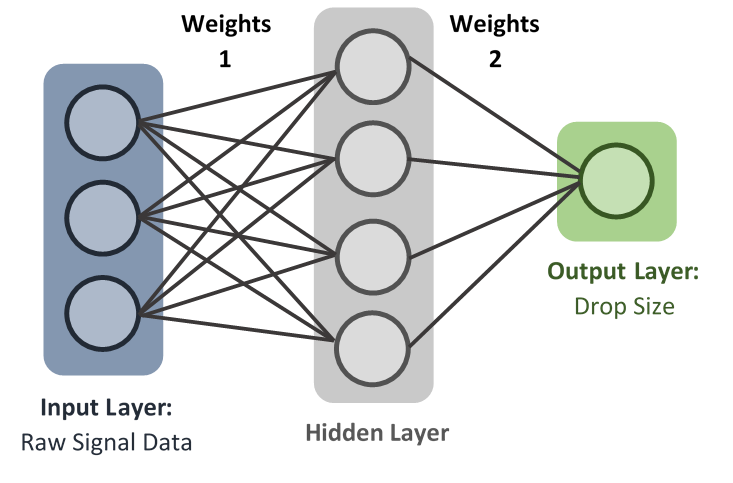Spray Analysis

# Applying Neural Networks to Spray Technology## 6

#### Aug 20

Neural networks are a set of algorithms, modeled loosely after the human brain, that are designed to recognize patterns. They are a mathematical function that map a given input to the desired output.Neural networks consist of an input layer, hidden layers, an output layer, a set of weights and biases between each layer, and an activation function for each hidden layer.

The values of the weights and biases determine the strength of the predictions within the model. The process of fine-tuning the weights and biases from the input data is known as training the neural network.

Each iteration of the training process calculates predicted outputs and updates each layer’s weights and biases. The weights and biases are updated based on ‘loss’ or ‘error’ in each layer; loss is the difference between each predicted value and the actual value. Our goal in training is to find the set of weights and biases that best minimizes the loss. Random weights are initially assigned to each layer, and the training process refines these weights until the error between the predicted values and the actual value is minimized.

## How are we using neural networks in spray technology?

We are using neural networks to predict the drop size of a spray based solely on signal data from a sensor. Using neural networks to predict the drop size values means the system’s estimation capabilities can be expanded to cover data for all sorts of chemicals, not just water. This is extremely beneficial because it makes the device’s development and implementation faster, cheaper, and easier to refine.

## Why do we need neural networks in spray technology?

Drop size measurement devices cost upwards of \$250,000, not including the costs of the highly trained technicians and engineers who collect and interpret the data. A mobile, neural- network-powered drop size measuring device would cost significantly less and could be operated by anyone with the software and some initial operating instructions and basic training. Depending on the application and the quality of the output data, the ROI on such an instrument could be measured in months, or even weeks.

May 17, 2022

May 16, 2022

April 7, 2022

March 7, 2022

March 4, 2022